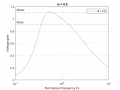# LTspice - need help tuning NCP1395 parameters.

#### boruwkarz

Joined Nov 19, 2019
9
Hello, I am designing LLC converter and I would like to control it with frequency modulation.
When desgining i followed this application note from infineon. I chose Q = 0.2 and m = 8.6 and here is the plot showing gain in function of normalized frequency (ratio of switching frequency to resonant frequency).I was looking for a long time for a way to modulate the frequency in order to compensate input voltage variation and finally I found this page with models and examples. I wanted to use that in my converter but all my attempts failed. I was following the example (hbllc_feedback_c.asc) and this application note.
I would like to get 100 V output working at 150 kHz (this is the resonant frequency). When I simulated the converter without this NCP1395 controller I was able to achieve that.
The parameters of the converter are:
Input voltage: 325 V DC (rectified and filtered 230 AC)
Maximum output power: 1 kW (100 V, 10 A)
Resonant frequency: 150 kHz

The voltage can be regulated by changing the frequency from 86 kHz (0.9 voltage gain) to 205 kHz (1.1 voltage gain).
Moving on to the problem. I've set the reference voltage and the feedback to many different levels, I tried to change parameters of the models from the NCP 1395 rectangle (on the schematic) (parameters: FMIN and FMAX) and parameters from the ampsimp block (OpAmp look alike the one on the right) (parameters: VHIGH and VLOW) and nothing did work properly. After the feedback voltage and reference voltage met at the same level, the feedback voltage kept rising and "error" signal dropped to zero, the switching frequency changed to 110 kHz and the output voltage stabilized much above 100 V (120, 130 V).Here are the plots of feedback voltage -blue colour (from voltage divider 100 V to 2.5 V), reference voltage - green colour, ampsimp output - red colour.
The plots on the left are from my simulation (LLC_1kW.asc) and the right one is from the example from ltwiki.org resources (hbllc_feedback_c.asc).

My question is: How to set the parameters of this three models (NCP 1395 - in the control loop) to maintain 100 V on the output and possibly at 150 kHz which is the most efficient point of operation.

If you need any more information about different parameters or anything feel free to ask or look in the LLC_1kW.asc file that I've attached.

#### Attachments

• 6.7 KB Views: 21
• 9 KB Views: 21

#### boruwkarz

Joined Nov 19, 2019
9
Ok, I solved the problem.

If anyone is interested. I switched feedback and voltage reference polarity on the "ampsemi" block.
I also modified frequency limits to Fmax = 230 kHz and Fmin = 110 kHz. Seems like the mathematical calculations as shown in the infineon appliaction note that I performed in MatLab don't translate 1:1 in LTspice simulation. Propably due to higher level of corectness in LTspice than in first harmonic approximation calculations.

If anyone got some other ideas why else that solved the problem or if anyone can prove me wrong, please do! I'd like to learn as much as I can.

Best regards everyone.